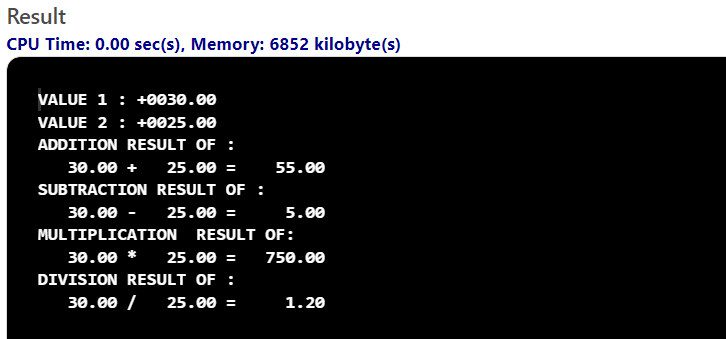# Display Computation in COBOL

• Last Updated : 02 Aug, 2022

DISPLAY is the most common form of internal data representation. DISPLAY stores in decimal form. Each character of the data will represent one byte of storage. If there is no usage clause for data items, then by default it will come under DISPLAY.

DISPLAY can be used for all types namely Numeric data types, Alphabetic data types, and Alpha-Numeric data types.

Syntax:

DISPLAY  NUMERIC/ALPHABETIC

• Numeric:     Represents all types of numerical variables and program values or results/outputs.
• Alphabetic: Represents String/texts, and special characters to explain or simplify the program to make it understandable to all.

Example Of Alphabetic Representation:

`DISPLAY "ADDITION RESULTS: "  `

Example Of Numeric Representation:

`DISPLAY  WS-VARIABLE1          `

Example Of Alpha-Numeric Representation:

```DISPLAY  "RESULTS OF "
WS-VAR1  "AND"   WS-VAR2
"IS"  WS-RESULT```

Example:

## Cobol

 `Cobol program for Display Computation.``IDENTIFICATION` `DIVISION``.``PROGRAM``-``ID``. Displays-Example.``DATA` `DIVISION``.``    ``WORKING-STORAGE` `SECTION``.``    ``01` `WS-NUM01 ``PIC` `S9(``4``)V99.``    ``01` `WS-NUM02 ``PIC` `S9(``4``)V99.``    ``01` `WS-VAR01 ``PIC` `9``(``4``)V99.``    ``01` `WS-VAR02 ``PIC` `9``(``4``)V99.``    ``01` `WS-RESULT ``PIC` `S9(``5``)V99 ``COMP``.``    ``01` `WS-RES01 ``PIC` `-ZZZ9.``99``.``    ``01` `WS-RES02 ``PIC` `-ZZZ9.``99``.``    ``01` `WS-RES03 ``PIC` `-ZZZ9.``99``.``    ``01` `WS-RES04 ``PIC` `-ZZZ9.``99``.``    ``01` `WS-VAR05 ``PIC` `-ZZZ9.``99``.``    ``01` `WS-VAR06 ``PIC` `-ZZZ9.``99``.``PROCEDURE` `DIVISION``.``    ``SET` `WS-NUM01 ``TO` `30``.``    ``SET` `WS-NUM02 ``TO` `25``.``MAIN-PARA.``    ``DISPLAY` `'VALUE 1 : '` `WS-NUM01.``    ``DISPLAY` `'VALUE 2 : '` `WS-NUM02.``    ``MOVE` `WS-NUM01 ``TO` `WS-VAR01.``    ``MOVE` `WS-NUM02 ``TO` `WS-VAR02.``    ``MOVE` `WS-NUM01 ``TO` `WS-VAR05.``    ``MOVE` `WS-NUM02 ``TO` `WS-VAR06.``    ``PERFORM` `ADDITION-PARA.``    ``STOP` `RUN``.``ADDITION-PARA.``    ``ADD` `WS-VAR01 WS-VAR02 ``GIVING` `WS-RESULT.``    ``MOVE` `WS-RESULT ``TO` `WS-RES01.``    ``PERFORM` `SUBTRACTION-PARA.``SUBTRACTION-PARA.``    ``SUBTRACT` `WS-VAR02 ``FROM` `WS-VAR01 ``GIVING` `WS-RESULT.``    ``MOVE` `WS-RESULT ``TO` `WS-RES02``    ``PERFORM` `MULTIPLICATION-PARA.``MULTIPLICATION-PARA.``    ``MULTIPLY` `WS-VAR01 ``BY` `WS-VAR02 ``GIVING` `WS-RESULT.``    ``MOVE` `WS-RESULT ``TO` `WS-RES03.``    ``PERFORM` `DIVISION``-PARA.``DIVISION``-PARA.``    ``DIVIDE` `WS-VAR01 ``BY` `WS-VAR02 ``GIVING` `WS-RESULT.``    ``MOVE` `WS-RESULT ``TO` `WS-RES04.``    ``PERFORM` `RESULT-PARA.``RESULT-PARA.``    ``DISPLAY` `'ADDITION RESULT OF : '``.``    ``DISPLAY` `WS-VAR05 ``' +'` `WS-VAR06 ``' = '``WS-RES01.``    ``DISPLAY` `'SUBTRACTION RESULT OF : '``.``    ``DISPLAY` `WS-VAR05 ``' -'` `WS-VAR06 ``' = '``WS-RES02.``    ``DISPLAY` `'MULTIPLICATION  RESULT OF: '``.``    ``DISPLAY` `WS-VAR05 ``' *'` `WS-VAR06 ``' = '``WS-RES03.``    ``DISPLAY` `'DIVISION RESULT OF : '``.``    ``DISPLAY` `WS-VAR05 ``' /'` `WS-VAR06 ``' = '``WS-RES04.`

Output:Explanation:

In this program, we are taking the two variables for all operations like addition, subtraction, multiplication, and division. Then we are displaying the proper heading using the DISPLAY keyword and also display the numerical results of the operation. We also tried to maintain proper code formatting using DISPLAY which helps to provide output in an understandable format.

My Personal Notes arrow_drop_up